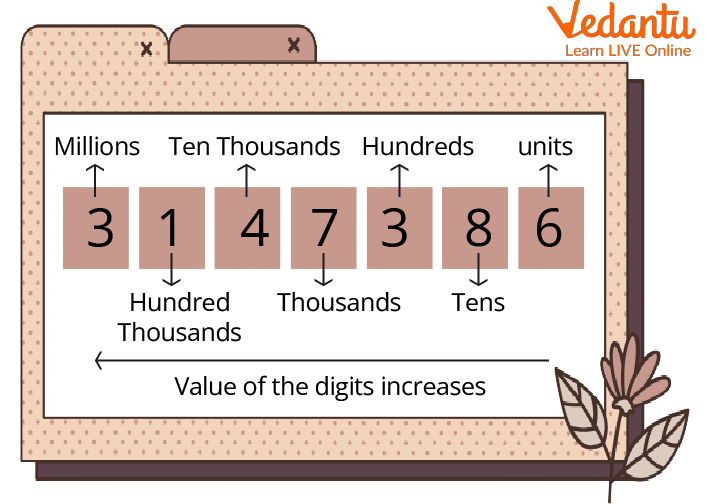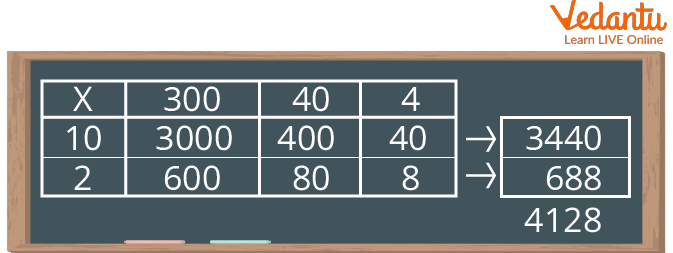Courses
Courses for Kids
Free study material
Offline Centres
More

# Expansion Method of MultiplicationLast updated date: 10th Dec 2023
Total views: 116.1k
Views today: 1.16k## Introduction to the Expansion Method

Let's start with an example: suppose you go to the market and buy 62 apples, and 1 apple costs 7 rupees. You have to calculate the rupees spent to buy 62 apples. Here, we can expand the number into two parts $60+2$. Now multiply $60 \times 7=420$ and $2 \times 7=14$, and the total rupees would be 434. So it's quite easy to multiply using the expansion method. In this article, we will learn about the expanded form multiplication and box method of multiplication. We will also solve some practice questions.

## What do You Mean by Expanded Form Multiplication?

The expanded form of multiplication is useful for splitting the higher-order digits and representing them in the form of units, 10s, 100s, and 1000s. Expanded forms help you better understand and read the top digits correctly. When solving multiplication problems using expanded form, you give each digit a value based on its position within the number.

## Writing Numbers in Expanded Form

A number expansion is the separation of numbers by their place values. This is an intermediate step that helps you understand how to read numbers. The expanded form is useful for knowing the number of digits in each number. Also, there are three different ways to write numbers in expanded form. The number 4537 is expanded in one form as $4537=4000+500+30+7$, in a second form as

$4531=4 \times 1000+5 \times 100+3 \times 10+7 \times$ 1.

And in the third form can be written as

$4537=4 \times \operatorname{thousand}(1000)+5 \times \operatorname{Hundred}(100)+3 \times \operatorname{ten}(10)+7 \times$ one (1).Placing Value in the Place Value Chart

## Expansion Method Multiplication

In the Expansion Method of Multiplication, we generally expand the number using expanded form into place value then we multiply the units and add them together.

For example, Multiply by expanding the bigger number, $25 \times 5$

We separate the number into two unit

20 (in Tens place value) and 5 (in one place value)

$20 \times 5=100$

$5 \times 5=25$

$100+25$ (Now add the number)

= 125

Thus the multiplication of 25 and 5 using an expanded form is 125.

## Box Method of Multiplication

The box method is another way to multiply two-digit and three-digit numbers. Instead of stacking numbers and remembering placeholders, the box method expands numbers and uses addition. The box method reduces multiplication to the basic ability of addition, without having to remember all the tedious rules that come with traditional multiplication.

## Box Method Formula and its Use

To use the box method for multiplication, we need to follow a few steps.

• Write both numbers in expanded form

• Draw a box based on the number of digits in each number

• Multiply each row and column of the box by the corresponding number

• Add the numbers in the box to get the final value.

The first step in using the box multiplication method is to write each number in expanded form. Writing numbers in expanded form means writing each number separated by various digits 1, 10, 100, etc. added together.

The image below shows exactly what this box will look like when fully set up. Multiply to fill individual boxes and then take the sum to obtain the corresponding product, i.e. 3440 + 688 = 4128.

Thus, the multiplication of 12 and 344 gives 4128.Box Method Calculation

## Solved Examples

Q 1. Multiply by expanding the bigger number, $902 \times 7$.

Ans: We separate the number into two units

900 (in Hundred place value), 00 (in tens place value), and 2 (in one place value)

Now, multiplying $900 \times 7=6300$

$00 \times 7=00$

and $2 \times 7=14$

$6300+00+14$ (Now add the number)

= 6314

Thus the multiplication of 902 and 7 using an expanded form is 6314.

Q 2. Multiply by expanding the bigger number using the box method for 34 and 5344.

Ans: Set the box to multiply 34 and 5344. Looking at the two numbers above, we can see that 34 has 2 digits and 5344 has 4 digits. You will need to set up $2 \times 4$ boxes. The image below shows exactly what this box will look like when fully set up. Multiply to fill individual boxes and then take their sum to obtain the multiplication of two numbers, i.e. $160320+21376=181696$

Thus, the multiplication of 34 and 5344 gives 181696.

## Practice Questions

A. Using Expansion Method:

Q 1.954 90.

Ans: 85860

Q 2. $769 \times 2$.

Ans: 1524

B. Using Box Method of Multiplication:

Q 1.5054 90.

Ans: 454860

Q 2. $769 \times 20$.

Ans: 15380

## Summary

In this article, we have learned about how to use the expanded form multiplication & expansion method to expand the biggest digit and put them into place with values of ones, tens, hundreds, and so on. We learned how to multiply them and take their sum to get the result of multiplication. We also learn about the box method of multiplication. It solves multiplication problems using a box, which is drawn taking into mind the number of digits. Some examples and practice problems are also given for clarity of concepts.

## FAQs on Expansion Method of Multiplication

1. Which formula is used to perform multiplication?

The formula that we use to perform multiplication is given:

'Multiplicand × Multiplier = Product.

2. How is multiplication related to addition?

Multiplication represents the basic idea of repeated addition of the same number. It simplifies the task of repeated addition. Repeated addition of a number is equivalent to the multiplication of the number with the number of repetitions.

3. What are the multiplication strategies?

Multiplication strategies are different ways in which multiplication can be learned. For example, multiplication using a number line, multiplication with the help of a place value chart, separating the Tens and Ones place and then multiplying them separately, and so on. These strategies help learners to understand the multiplication concept with a broader perspective.